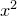# 5.5 Tabulating functions and slicing data files

Pyxplot’s tabulate command is similar to its plot command, but instead of plotting a series of data points onto a graph, it writes them to a data file. It can be used to produce text files containing samples of functions, to rearrange/filter the columns in data files, to produce a copy of a data file using different physical units, and so on.

The following example would produce a data file called gamma.dat containing a list of values of the gamma function:

```set output 'gamma.dat'
tabulate [1:5] gamma(x)
```

One way to tabulate multiple functions into a common file is with the using modifier, as in the example

```tabulate [0:2*pi] sin(x):cos(x):tan(x) using 1:2:3:4
```

This tabulates the supplied functions horizontally alongside one another in a series of columns. As many expressions may be supplied to the using modifier as columns are wanted.

Alternatively, if a series on functions or data files are listed in a comma-separated list (as is done in the plot command to plot multiple datasets), the functions are tabulated one after another in a series of index blocks separated by double linefeeds (see Section 3.8):

```tabulate [0:2*pi] sin(x), cos(x), tan(x)
```

The set samples command can be used to control the number of points that are listed when tabulating functions, in the same way that it controls the number of data points drawn by the plot command:

```set samples 200
```

If the abscissa axis is set to be logarithmic then the functions are evaluated at logarithmically-space points along the axis; otherwise, they are samples at linearly-spaced points.

The select, using and every modifiers operate in the same manner in the tabulate command as in the plot command. Thus the following example would write out the third, sixth and ninth columns of the data file input.dat, but only when the arcsine of the value in the fourth column is positive:

```set output 'filtered.dat'
tabulate 'input.dat' using 3:6:9 select (asin(\$4)>0)
```

The numerical display format used for each column of the output file is automatically chosen to preserve accuracy whilst simultaneously being as easily human readable as possible. Thus, columns which contain only integers are displayed as such, and scientific notation is only used in columns which contain very large or very small values.

If desired, however, a custom format may be specified using the with format modifier. This can be used both to specify text to appear in between the columns of data, and to specify the format of the data itself using tokens such as %.5f, as used by Pyxplot’s string substitution operator (%; see Section 6.2.1), and the sprintf statement of many other programming languages.

For example, to tabulate the values ofto very many significant figures with some additional text, one could use:

```tabulate x**2 with format "x = %f ; x**2 = %27.20e"
```

This might produce the following output:

```x = 0.000000 ; x**2 =  0.00000000000000000000e+00
x = 0.833333 ; x**2 =  6.94444444444442421371e-01
x = 1.666667 ; x**2 =  2.77777777777778167589e+00
```

There is flexibility as to how many substitution tokens appear in the format specification. If the number of tokens is fewer than the number of columns of data, then the format is repeated until all the columns have been printed. Thus, the command

```tabulate x**2 with format "%.3f "
```

might produce the output:

```0.000 0.000
0.833 0.694
1.667 2.778
```

Note that the space character at the end of the format is important to ensure that there is a gap between the columns.

If formats are supplied for more columns than are present, then the final columns are padded with nan (not a number).

The data produced by the tabulate command can be sorted in order of any arbitrary metric by supplying an expression after the sortby modifier. The data are sorted in order from the lowest value of this expression to the highest.